### 2.1.4 Periodic Potentials and Bloch's TheoremIn the most simplified version of the free electron gas, the true three-dimensional potential was ignored and approximated with a constant potential (see the quantum mechanics script as well) conveniently put at 0 eV.The true potential, however, e.g. for a Na crystal, is periodic and looks more like this (including some electronic states):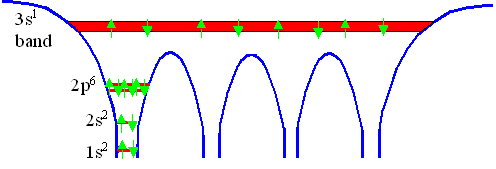Semiconducting properties will not emerge without some consideration of the periodic potential – we therefore have to solve the Schrödinger equation for a suitable periodic potential. However, this is much easier said than done: There are several ways to do this (for real potentials always numerically), but they are all mathematically rather involved – and, thus, beyond the scope of this lecture.Luckily, as stated before, it can be shown that all solutions must have certain general properties. These properties can be used to make calculations easier – as well as to obtain a general understanding of the the effects of a periodic potential on the behavior of electron waves.The starting point is a potential V(r) determined by the crystal lattice that has the periodicity of the lattice, i.e.
V(r)  =  V(r + T)With T = any translation vector of the lattice under consideration.We then will obtain some wavefunctions y(r) which are solutions of the Schrödinger equation for V(r). In addition, these wavefunctions have to fulfill the boundary conditions, since we are still dealing with a kind of "particle in a box" problem – the electrons are confined inside the crystal.

The Bloch TheoremThe Bloch theorem in essence formulates a condition that all solutions y(r), for any periodic potential V(r) whatsoever, have to meet. In one version it ascertains
y(r)  =  u(r) · exp (i · k · r)With k = any allowed wave vector for the electron that is obtained for a constant potential, and u(r) = some functions with the periodicity of the lattice, i.e.
u(r + T)  =  u(r)Any wavefunction meeting this requirement we will henceforth call a Bloch wave.As before, we choose periodic boundary conditions; this ensures that no restiction on the translation vectors T needs to be considered.The Bloch theorem is quite remarkable, because, as said before, it imposes very special conditions on any solution of the Schrödinger equation, no matter what the form of the periodic potential might be.We notice that, in contrast to the case of the constant potential, so far, k is just a wave vector in the plane wave part of the solution. Due to the periodic potential, however, its role as an index to the wave function is not the same as before – as we will shortly see.Bloch's theorem is a proven theorem with perfectly general validity. We will first give some ideas about the proof of this theorem and then discuss what it means for real crystals. As always with hindsight, Bloch's theorem can be proved in many ways; the links give some examples. Here we only look at general outlines of how to prove the theorem:It follows rather directly from applying group theory to crystals. In this case one looks at symmetry properties that are invariant under translation.It can easily be proved by working with operator algebra in the context of formal quantum theory mathematics (see the quantum mechanics script again).It can be directly proved in simple ways – but then only for special cases or with not quite kosher "tricks".It can be proved (and used for further calculations) by expanding V (r) and y(r) into a Fourier series and then rewriting the Schrödinger equation. This is a particularly useful way because it can also be used for obtaining specific results for the periodic potential. This proof is demonstrated in detail in the link, or in the book of Ibach and Lüth.Bloch's theorem can also be rewritten in a somewhat different form, giving us a second version:
y (r + T)  =  y (r) · exp(ikT)This means that any function y(r) that is a solution to the Schrödinger equation of the problem, differs only by a phase factor exp(ikT) between equivalent positions in the lattice.This implies immediately that the probability of finding an electron is the same at any equivalent position in the lattice, exactly as we expected, because
|y(r + T)|2  =  |y(r )|2  ·  |exp(ikT)| 2  =  |y (r)|2This is so because |exp(ikT)|2 = 1 for all k and T.That this second version of Bloch's theorem is equivalent to the first one may be seen as follows:If we write the wave function in the first form y (r) = u(r) · exp(ikr) and consider its value at an equivalent lattice position r + T we obtain
y (r + T)  =  u(r + T)  · exp [ik · (r + T)]    =  u( r) · exp (ikr)  · exp (ikT)   =  y(r ) · exp(ikT)

=  u(r )     =  y (r)     q.e.d.Bloch's theorem has many more forms and does not only apply to electrons in periodic potentials, but for all kinds of waves in periodic structures, e.g. phonons. However, we will now consider the theorem to be proven and only discuss some of its implications.Most importantly, we will now clarify what index to apply to the wave function y(r) and, thus, also to its lattice-periodic part u(r).

Implications of the Bloch TheoremOne difference to the constant potential case is most crucial: If we know the wavefunction for one particular k value, we also know the wavefunctions for infinitely may other k values, too.This follows from the fact that in the "second version" of the Bloch theorem, y(r + T) = y(r) · exp(ikT), the vector k is not unique, since for any reciprocal lattice vector g it holds that exp(igT) = 1. Hence, when k is replaced by k' = k + g, the Bloch theorem is also fulfilled:
y(r + T)  =  y(r) · exp[i(k + g) T]  =  y ( r) · exp(ikT)Obviously it is not clear which reciprocal space vector, k or k', is to be associated with this Bloch wave; this is why the index k was omitted so far. (That this non-uniqueness also holds for u(r) can be seen from its Fourier series representation; cf. the relevant proof of the Bloch theorem.)The deeper reason behind this property is that, similar to E being an eigenvalue of the Hamilton operator H in the equation Hy = Ey , the exponential expressions exp(ikT) are the eigenvalues of the translation operator T in the equation Ty = exp(ikT)y (cf. the relevant proof of the Bloch theorem), and these eigenvalues do not change when k is replaced by k' = k + g.Therefore, in principle not a single vector k is to be attributed as an index to a specific wavefunction, but all k' = k + g.All these points in k-space are equivalent, because for any reciprocal lattice vector g it holds that yk + g(r) = yk (r).On the other hand, this permits to restrict the k vector to the first Brillouin zone, because any k' not in the first Brillouin zone can always be written as k' = k + g, with k now being in the first Brillouin zone.Keeping this in mind, we can now simply write y k(r); further implications are discussed below.In general, a Bloch wave yk(r) = uk(r) · exp(ikr) can be understood as a lattice-peridocally modulated plane wave.One way of looking at this "first version" of the Bloch theorem is to interprete the lattice-periodic function uk(r) as a kind of correction factor that is used to generate solutions for periodic potentials from the simple solutions for constant potentials.We then have good reasons to assume that uk(r) for k vectors not close to a Brillouin zone edge will only be a minor correction, i.e. uk( r) should be close to 1, then.But in any case, the quantity k, while still being the wave vector of the plane wave that is part of the Bloch wave function (and which may be seen as the "backbone" of the Bloch functions), has lost its simple meaning: Besides not being unique, there are explicit reasons why it can no longer be taken as a direct representation of the momentum p of the electron via p =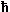k, or of its wavelength l = 2p/k:The momentum of the electron moving in a periodic potential, having the wave function yk(r) = uk(r) · exp(ikr), can be calculated from –i
yk( r), thus it is not only related to k but also touk(r), which represents the influence of the lattice. As an example, for the standing waves resulting from (multiple) reflections at the Brillouin zone edges the momentum of the electron is actually zero (because the velocity is zero), while k is not.There is no unique wavelength to a plane wave modulated with some arbitrary periodic function. Its Fourier decomposition can have any spectrum of wavelengths, so which is the one to be relevant for being associated with k?To make this clear, sometimes the vector k for Bloch waves is called the "quasi wave vector".Nevertheless, k is a quantum number related to the translational symmetry of the lattice. Thus, instead of associating it with the momentum of the electron which is related to the translational symmetry of free space, we may identify the quantityk with the so-called crystal momentum P, being relevant for the movement of the electron in the periodic lattice.The crystal momentum P is something like the combined momentum of crystal and electron. While not being a "true" momentum (which should be expressible as the product of a distinct mass and a velocity), it still has many properties of momenta, in particular it is conserved during all kinds of processes (as we will see later on).This is a major feature for the understanding of semiconductors, as we will see soon enough!Only if V = 0, i.e. if there is no periodic potential, then the crystal momentum is equal to the bare electron momentum; i.e. then the part of the crystal is zero.

Reduced- and Repeated-Zone SchemesWe now consider the far-reaching consequences of the fact that yk + g(r ) = yk(r) for the energy value(s) associated with that wavefunction; with g = arbitrary reciprocal lattice vector.Writing this as y(k + g, r) = y(k, r) and taking k as a continuous variable, this means that y(k , r) is periodic in k-space.As a solution of the Schrödinger equation for the system, associated with y(k , r) there is a specific energy E( k) which necessarily is also periodic:
E(k + g)  =  E( k)This is a major insight; it means that any reciprocal lattice point can serve as the origin of the E(k) function.Let's visualize this for the case of an infinitesimaly small periodic potential – we have the periodicity, but not a real potential. The E(k) function then is practically the same as in the case of free electrons, but starting at every point in reciprocal space: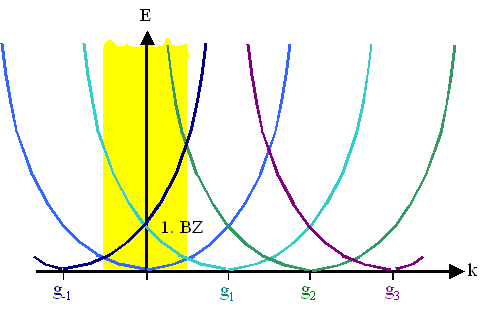As a consequence, we now have many energy values for one given k, and in particular all possible energy values are contained within the first Brillouin zone, i.e. between –0.5g1 and +0.5g1 in the picture, which in total is an example of a repeated-zone scheme because the same holds for all Brillouin zones.It thus is sufficient to consider only the first Brillouin zone in graphical representations of the E(k) function – it contains all the information available about the system.This is called a reduced representation of the band diagram (or reduced-zone scheme), which may look like this: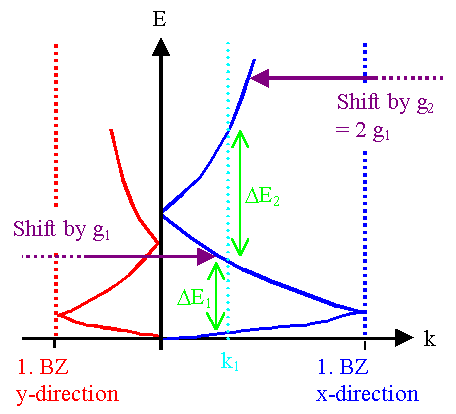The branches outside the 1. BZ have been shifted ("folded back") into the 1. BZ, i.e. translated by the appropriate reciprocal lattice vector g.To make band diagrams like this one as comprehensive as possible, the symmetric branch on the left side is omitted; instead, the band diagram in a different direction in reciprocal space is shown.Altogether, this now looks like a specific electron (i.e., with a specific k) could have many energies all at once – which is, of course, not the case .Different energies, formerly distinguished by different k-vectors, are still different energies, but in the first Brillouin zone they are distinguished by considering them to form different bands .Every energy branch coming from larger k-vectors carries an index n denoting the band ; this index specifies the original Brillouin zone that this branch originated from.Formally, in the first Brillouin zone we have the function En (k) associated with the Bloch wave yn,k(r ) .The identical construction, but now for the energy functions of a periodic potential as given before, now looks like this: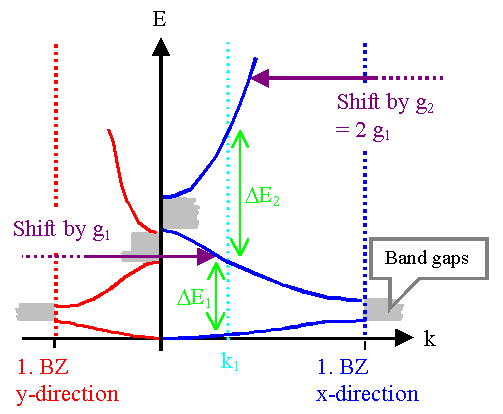We now have band gaps – regions with unattainable energies – in all directions of the reciprocal lattice.A numerical example for the Kroning–Penney Model is shown in this link.What does this mean for a particular electron, say one on the lowest branch of the blue diagram with the wave vector k1? It has a definite energy E associated with it.But it also could have larger energies – all the values obtained for the same k but in higher branches of the band diagram.For a transition to the next higher branch the energy DE1 is needed. It has to be supplied from the outside world.After the transition the electron has now a higher energy, but the wave vector is the same. But wait, in the reduced band diagram, we simply omitted a reciprocal wave vector, so its wave vector is actually k1 + g. If we index the situation after the transition with "2", before with "1", we have the following equations:
E2  =  E1 + DE

k2  =  k1 + g

|k1|   ¹  |k2|This is simply Braggs law, but now for inelastic scattering, where the magnitude of k may change – but only by a specified amount tied to a reciprocal lattice vector.Since we interpretedk as crystal momentum , we may consider Braggs law to be the expression for the conservation of momentum in crystals.The reduced band diagram representation thus provides a very simple graphical representation of allowed transitions of electrons from one state, represented by (E1, k1), to another state (E2 , k2 ): the states must be on a vertical line through the diagram, i.e. straight up or down.An alternative way of describing the states in the spirit of the reduced diagram is to use the same wave vector k for all states and a band index for the energy. The transition then goes from (En, k) to (Em, k ) with n, m = number of the energy band involved.The possibility of working in a reduced band diagram, however, does not mean that wave vectors larger than all possible vectors contained in the 1. BZ are not meaningful or do not exist:Consider an electron "shot" into the crystal with a high energy and thus a large k – e.g. in an electron microscope. If you reduce its wave vector by subtracting a suitable large g vector without regard to its energy and band number, you may also reduce its energy – you move it, e.g., from a band with a high band number to a lower one. While this may happen physically, it will only happen via many transitions from one band to the next lower one – and this takes time!Most of the time in normal applications the electron will keep its energy and its original wave vector. And it is this wave vector you must take for considering diffraction effects! An Ewald (or Brillouin) construction for diffraction will give totally wrong results for reduced wave vectors – think about it!If you feel slightly (or muchly) confused at this point, that is as it should be. Bloch's theorem, while relatively straightforward mathematically, is not easy to grasp in its implications to real electrons. The representation of the energy–wave vector relationship (the dispersion curves) in extended or reduced schemata, the somewhat unclear role of the wave vector itself, the relation to diffraction via Bragg's law, the connection to electrons introduced from the outside, e.g. by an electron microscope (think about it for minute!), and so on, are difficult concepts not easily understood at the "gut level".While it never hurts to think about these questions, it is sufficient for our purpose to just accept the reduced band structure scheme and its implications as something useful in dealing with semiconductors – never mind the "small print" associated with it.However, if you want to dig deeper: All these effects that are difficult to grasp are to some extent rooted in the formal quantum mechanics behind Bloch's theorem. It has to do with eigenvectors, eigenvalues and commutation of operators; so, if you know about that, all these effects come rather naturally.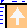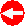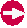© H. Föll (Semiconductors - Script)